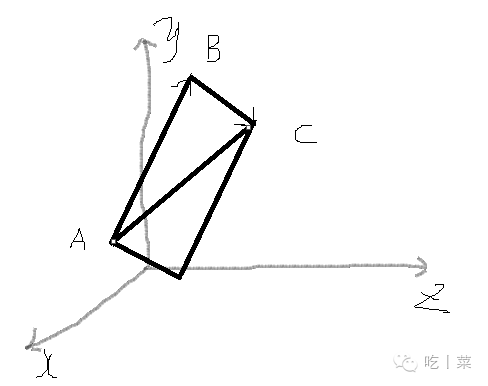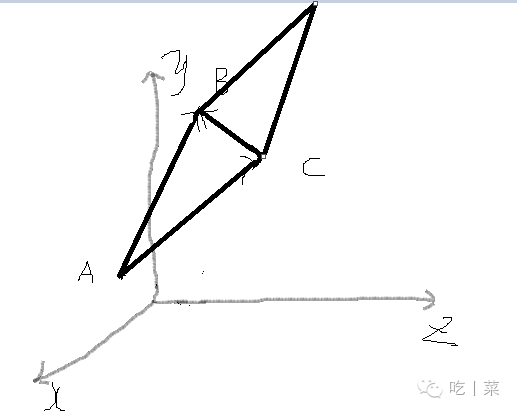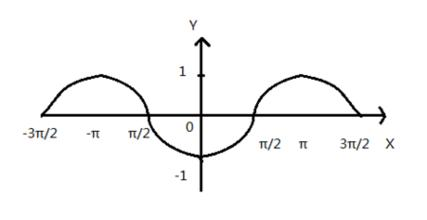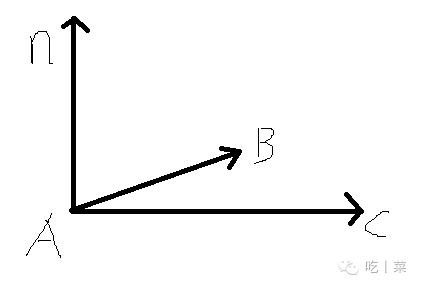# 3D数学-向量

• A+

A（1,1,2），B（3,6，1），C（1,5,3）

AB + BC = (2-2，5-1，-1+2) = (0，4，1) = ACAB = （2,5,-1），AC = (0，4，1), CB = (2，1，-2)

AB - AC = (2-0，5-4，-1-1) = (2，1，-2) = CBAB（单位向量） = （2/|AB|,5/|AB|,(-1)/|AB|）

AB = （2,5,-1），AC = (0，4，1)

AB · AC = 2×0 + 5×4 + （-1）×1 = 19AB = （2,5,-1），AC = (0，4，1)

AB × AC = (5×1-4×-1,-1×0-1×2,2×4-0×5) = (9,-2,8)

AB ·  (9,-2,8) = 2×9 + 5×（-2）+ （-1）×8 = 0

AC·  (9,-2,8) = 0×9 + 4×（-2）+ 1×8 = 0• 我的微信
• 扫一扫加我微信
•• 微信我的
• 我微信扫一扫加
•